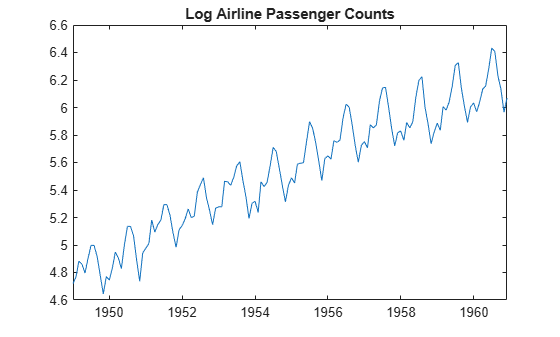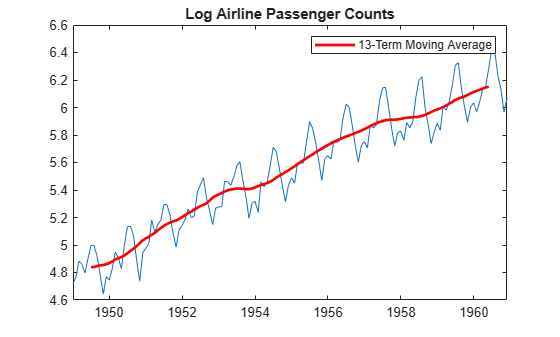# Moving Average Trend Estimation

This example shows how to estimate long-term trend using a symmetric moving average function. This is a convolution that you can implement using `conv`. The time series is monthly international airline passenger counts from 1949 to 1960.

Load the airline data set (`Data_Airline`).

```load Data_Airline y = log(DataTimeTable.PSSG); T = length(y); figure plot(DataTable.Time,y) title 'Log Airline Passenger Counts'; hold on```The data shows a linear trend and a seasonal component with periodicity 12.

The periodicity of the data is monthly, so a 13-term moving average is a reasonable choice for estimating the long-term trend. Use weight 1/24 for the first and last terms, and weight 1/12 for the interior terms. Add the moving average trend estimate to the observed time series plot.

```wts = [1/24; repmat(1/12,11,1); 1/24]; yS = conv(y,wts,'valid'); h = plot(DataTimeTable.Time(7:end-6),yS,'r','LineWidth',2); legend(h,'13-Term Moving Average') hold off```When you use the shape parameter `'valid'` in the call to `conv`, observations at the beginning and end of the series are lost. Here, the moving average has window length 13, so the first and last 6 observations do not have smoothed values.